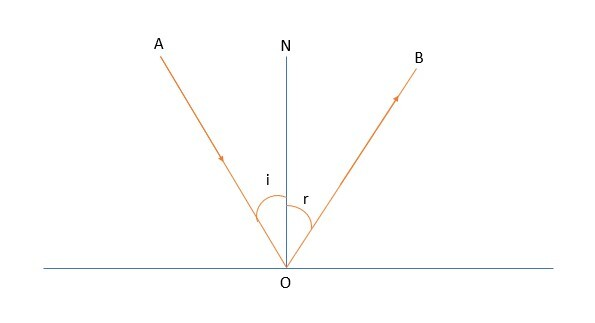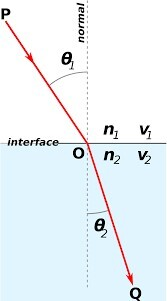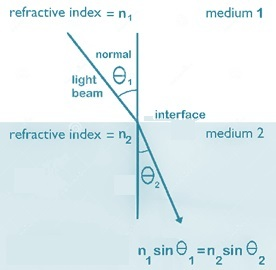# Angle of Incidence - Definition, Formula, Diagram

## Introduction

Angle of incidence and its measurement is a key concept of Optics. Light rays when incident on the surface like walls and mirrors, will reflect and get dispersed in the environment. The science of optics studies the traversing of light and its changing characteristics when passing from one medium to another medium. The terms like an incident ray, reflected ray, refracted rays, incident angle refracted angle and reflection, refraction index, and like terms clearly articulate the light characteristics which benefit us in many ways. This article is an attempt to describe the reflection and refraction phenomenon of light and its relative principles. The common medium in which light travel are air, water, glass, diamond, etc. The characteristics of light in different materials as above are also explained using standard laws and equations.

## What is the Angle of Incidence?

Two angles are formed at the extremity of incidence, when beam of light fall on a surface. The angle formed by source ray with the normal, is called incidence angle and the angle formed by the reflected ray with normal is called reflected angle. In optical physics, the imaginary line drawn at 90 degrees to the surface is called normal and you come across the term “normal” in many contexts. As per law of reflection, both angles are always identical.

## Concept of Light

Light is a form of energy. Light itself is invisible like other forms of energy but it renders all bodies both luminous and non-luminous objects visible. An object in a perfectly dark room conclusively establishes the truth that light is necessary for the purpose of vision.

## The Angle of Incidence Formula

The formula for angle of incidence in relation with angle of reflection is that both these angles are equal. So, if $\mathrm{\theta_i}$ is incidence angle and if $\mathrm{\theta_r}$ is reflection angle, then

$$\mathrm{\theta_i=\theta_r}$$

Normal observations state the angle of light rays to the surface. For example, if the angle between a ray to surface is measured as 20°, then angle of incidence is 90° - 20° = 70°. And hence angle of reflection is also 70°.

### What is the relationship between the Angle of Incidence and the Angle of Reflection?

• From image we observe that an incident ray, reflected ray and the normal. Incident ray is AO, reflected ray is OB and normal is NO. O is the point of incidence.

• The marked ‘i’ is the incident angle and ‘r’ is the reflected angle.

• Incident angle ‘i’ = reflected angle ‘r’

• The incident ray and reflected ray are on the same point (o).## The Angle of Incidence and Angle of Reflection

Light rays either gets absorbed or reflected or gets transmitted based on the type of surface it falls. When the surface is opaque, light rays gets reflected. Due to this reflection, the reflected ray forms an angle with normal which is called angle of reflection. The angle setup by incident ray with normal is an incidence angle. A portion of the incident ray of light which has the matching frequency of the electrons of atoms of the material of the surface is absorbed by the surface and never released as light energy. This absorbed energy turns to heat energy. Since the surface we are describing in the context of reflection, is not transparent, but opaque, the light energy never gets transmitted through the surface. Only reflection and absorption happen when light falls in an opaque medium or surface.

## The Angle of Incidence and Angle of Refraction

When a light beam travelling from a medium 1, falls obliquely on the surface of another medium, medium 2, then as it enters the medium 2, it undergoes an abrupt change in direction. The rays either go away from the normal in the other medium or bend towards the normal in the other medium. These rays are called refracted rays and these form an angle with normal and is called angle of refraction. The incidence angle is same as in case of reflection.In the above figure, $\mathrm{n_1}$and $\mathrm{n_2}$ are the refractive indexes of two mediums. $\mathrm{\theta_1}$ is incidence angle and $\mathrm{\theta_2}$ is refracted angle. The velociy of rays in first medium (air) $\mathrm{n_1}$ is $\mathrm{v_1}$ and velocity in second medium(water) $\mathrm{n_2}$ is $\mathrm{v_2}$. The angle of incidence is in medium $\mathrm{n_1}$ and angle of refraction is in medium $\mathrm{n_2}$.

## Difference between Angle of Incidence and Angle of Refraction

Refraction happens when crossover surface medium is transparent. Only a transparent surface of destination medium can allow the rays to pass through and thus allowing refraction. For example, if air is the source medium where light rays are originating and water is the second medium where light rays refract into from air medium, then water surface is acting as the crossover medium and is transparent. So light rays slightly bend in direction due to the refractive index of water. The small difference was observed by scientists whenever light rays pass from one medium to another transparent medium which allows the beam rays to pass through. This difference is best captured in the formula below and is called Snell’s law

$$\mathrm{\frac{N_1}{N_2}=\frac{Sin\theta_2}{Sin\theta_1}}$$Considering a single colour of light and for the two specific mediums in consideration, the proportion of sine of incidence angle to the sine of refracted angle will be always equal to the proportion of indices of refraction of the two mediums.

## Conclusion

The topic of light parameters in different mediums are discussed in detail with the help of diagrams and equations. Snell’s law is one of the important laws in optical physics, which define the index of refraction which is a constant. In reflection, the incidence and reflected angle is same whereas in refraction, the incidence and refracted angle is different, except when both mediums are same. The reversibility of light is an interesting phenomenon, where if we reverse the path of incidence and refraction, the light follows the same pattern as the initial pattern.

## FAQs

Q1. Why is that the angle of incidence needs to be computed when addressing real life scenarios?

Ans. It is easy and accurate to measure the angle of light rays to the surface. Once this value is observed, we need to subtract from the normal (90 degree) to get the angle of incidence. Hence many a times, we face situations where angle of incidence is computed from the observed surface angle.

Q2. What is the refractive index of air?

Ans. The Refractive index of air is 1.0003. In a vacuum, the refractive index is exact 1. Light travels slightly slower in the air than vacuum (space) and hence the index is slightly higher compared to that in a vacuum.

Q3. How is bending of light and speed of rays related to the refractive index?

Ans. During refraction, the speed of light increase in destination medium with lower refractive index. The rays in this situation will bend away from normal. The converse is true in a higher index destination medium.

Q4. When will the incidence angle is equal the refracted angle?

Ans. When the refractive index of two mediums is the same, then the incidence angle will be equal to the refracted angle.

Q5. How is the index of refraction calculated for a new medium which passes light?

Ans. We know the speed of light is a constant. Let c be speed of light beam in the new medium be v.

Now the index of refraction, usually denoted as n is calculated by the formula

$$\mathrm{n=\frac{ u}{c}}$$

Updated on: 28-Apr-2023

355 Views